Courses

# Olympiad Test: Odd One Out - 2

## 10 Questions MCQ Test Science Olympiad Class 7 | Olympiad Test: Odd One Out - 2

Description
This mock test of Olympiad Test: Odd One Out - 2 for Class 7 helps you for every Class 7 entrance exam. This contains 10 Multiple Choice Questions for Class 7 Olympiad Test: Odd One Out - 2 (mcq) to study with solutions a complete question bank. The solved questions answers in this Olympiad Test: Odd One Out - 2 quiz give you a good mix of easy questions and tough questions. Class 7 students definitely take this Olympiad Test: Odd One Out - 2 exercise for a better result in the exam. You can find other Olympiad Test: Odd One Out - 2 extra questions, long questions & short questions for Class 7 on EduRev as well by searching above.
QUESTION: 1

### Directions: In each problem, out of the five figures marked (1), (2), (3), (4) and (5), four are similar in a certain manner. However, one figure is not like the other four. Q. Choose the figure which is different from the rest.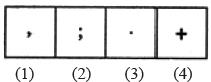Solution:

All others are punctuation marks.

QUESTION: 2

### Directions: In each problem, out of the five figures marked (1), (2), (3), (4) and (5), four are similar in a certain manner. However, one figure is not like the other four. Q. Choose the figure which is different from the rest.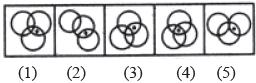Solution:

Only in fig. (4), the dot appears in the region common to all the three circles.

QUESTION: 3

### Directions: In each problem, out of the five figures marked (1), (2), (3), (4) and (5), four are similar in a certain manner. However, one figure is not like the other four. Q. Choose the figure which is different from the rest.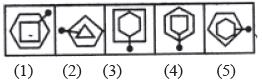Solution:

Only in fig. (4), the pin passes through a vertex of each one of the two elements.

QUESTION: 4

Directions: In each problem, out of the five figures marked (1), (2), (3), (4) and (5), four are similar in a certain manner. However, one figure is not like the other four.

Q. Choose the figure which is different from the rest.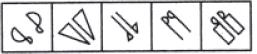Solution:

All other figures contain a geometrical figure along with its mirror image.

QUESTION: 5

Directions: In each problem, out of the five figures marked (1), (2), (3), (4) and (5), four are similar in a certain manner. However, one figure is not like the other four.

Q. Choose the figure which is different from the rest.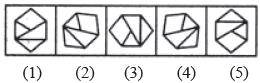Solution:

All other figures can be rotated into each other.

QUESTION: 6

Directions: In each problem, out of the five figures marked (1), (2), (3), (4) and (5), four are similar in a certain manner. However, one figure is not like the other four.

Q. Choose the figure which is different from the rest.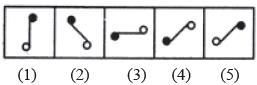Solution:

Figure (c) is different from other figures.

QUESTION: 7

Directions: In each problem, out of the five figures marked (1), (2), (3), (4) and (5), four are similar in a certain manner. However, one figure is not like the other four.

Q. Choose the figure which is different from the rest.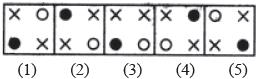Solution:

In each one of the figures except fig. (3), the two crosses (x) appear in the diagonally opposite corners.

QUESTION: 8

Directions: In each problem, out of the five figures marked (1), (2), (3), (4) and (5), four are similar in a certain manner. However, one figure is not like the other four.

Q. Choose the figure which is different from the rest.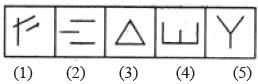Solution:

Figure (4) is formed by four line segments while each one of the other figures is formed by three line segments.

QUESTION: 9

Directions: In each problem, out of the five figures marked (1), (2), (3), (4) and (5), four are similar in a certain manner. However, one figure is not like the other four.

Q. Choose the figure which is different from the rest.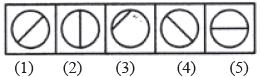Solution:

Only in fig. (3), the line segment is not a diameter of the circle.

QUESTION: 10

Directions: In each problem, out of the five figures marked (1), (2), (3), (4) and (5), four are similar in a certain manner. However, one figure is not like the other four.

Q. Choose the figure which is different from the rest.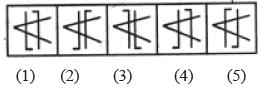Solution:

Only in fig. (4), both the parallel lines are bent in the same direction {i.e. towards the left).